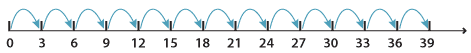### Simple multiplication

The basis of all multiplication is the ability to multiply single-digit numbers. For most of us, our first experience of multiplication was learning about multiples. Skip counting by a particular number gives us the multiples of that number. For example, the multiples of 3 are 3, 6, 9, 12, 15, 18, 21 and so on, as shown on the number line below.We can show multiplication using an array of objects. This array of stars shows 3 × 5 = 15.You should be fluent with multiplication up to 12 × 12 as shown in the following multiplication table. Fluency in this case means the ability to instantly recall these multiplications.
In this multiplication table the shaded numbers show the multiples of 4 up to 12 × 4.

× 0 1 2 3 4 5 6 7 8 9 10 11 12
0 0 0 0 0 0 0 0 0 0 0 0 0 0
1 0 1 2 3 4 5 6 7 8 9 10 11 12
2 0 2 4 6 8 10 12 14 16 18 20 22 24
3 0 3 6 9 12 15 18 21 24 27 30 33 36
4 0 4 8 12 16 20 24 28 32 36 40 44 48
5 0 5 10 15 20 25 30 35 40 45 50 55 60
6 0 6 12 18 24 30 36 42 48 54 60 66 72
7 0 7 14 21 28 35 42 49 56 63 70 77 84
8 0 8 16 24 32 40 48 56 64 72 80 88 96
9 0 9 18 27 36 45 54 63 72 81 90 99 108
10 0 10 20 30 40 50 60 70 80 90 100 110 120
11 0 11 22 33 44 55 66 77 88 99 110 121 132
12 0 12 24 36 48 60 72 84 96 108 120 132 144

Multiplication is commutative. It does not matter in which order we multiply, we get the same result. For example, the array of stars earlier shows 3 × 5 = 15 and 5 × 3 = 15. On the multiplication chart above, we might think that there are 144 facts to learn, but there are less because of this commutative property for multiplication.

There are a number of useful strategies for multiplication. Many of these can be done mentally or are useful pencil and paper strategies. As with addition and subtraction, you will need to begin assessing strategies to select the one that best serves your purpose. The numbers involved may dictate the best strategy to use. Many of the strategies can be learned with small numbers and extended for use with large numbers.

#### Multiplying by 9

If we want to get 9 lots of something, we can find 10 lots and then take 1 lot away. For example:

\begin{align} 9\times72&=10\times 72-72\\\\ &=720-72\\\\ &=648 \end{align}

#### Multiplying by 11

If we want to get 11 lots of something, find 10 lots and then add 1 lot more. For example:

\begin{align} 11\times72&=10\times 72+72\\\\ &=720+72\\\\ &=792 \end{align}

#### Multiplying by 5

The strategy for multiplying a number by 5 relies on knowing that 10 = 2 × 5. There are two ways to do it, depending on the number that you start with.

The first way is to halve the number, then multiply the result by 10. This method is best used when the number is easily halved. For example:

\begin{align} 48\times5&=24\times 10\\\\ &=240 \end{align}

The second way is to multiply by 10, then halve the result. For example:

\begin{align} 47\times5&=47\times10\div 2\\\\ &=470\div 2\\\\ &=235 \end{align}

#### Multiplying by 6

To multiply a number by 6, multiply the number by 3, then multiply it by 2. Or multiply by 2 first and then by 3. This works because 6 = 3 × 2. For example:

\begin{align} 52\times 6&=52\times 3\times 2\\\\ &=156\times 2\\\\ &=312 \end{align}

#### Halve and double

This technique involves moving a factor from one number to another in order to simplify a calculation. We halve one side of the multiplication and double the other. We keep doing this until we get a multiplication that is easier to handle mentally. For example:

\begin{align} 36\times 5&=18\times (2\times 5)\\\\ &=18\times 10\\\\ &=180 \end{align}

and

\begin{align} 64\times 24&=32\times 48\\\\ &=16\times 96\\\\ &=8\times 192\\\\ &=4\times 384\\\\ &=2\times 768\\\\ &=1536 \end{align}

#### Multiplying by any number

In fact the multiplying by 6 strategy can be modified so that we split any number we are multiplying by. To multiply by 12 we can multiply by 4 and then by 3. This works because 12 = 4 × 3. For example:

\begin{align} 25\times 12&=25\times 4\times 3\\\\ &=100\times 3\\\\ &=300 \end{align}

and

\begin{align} 150\times 14&=150\times 2\times 7\\\\ &=300\times 7\\\\ &=2100 \end{align}